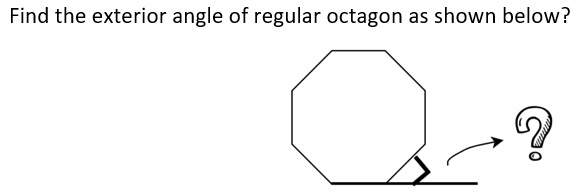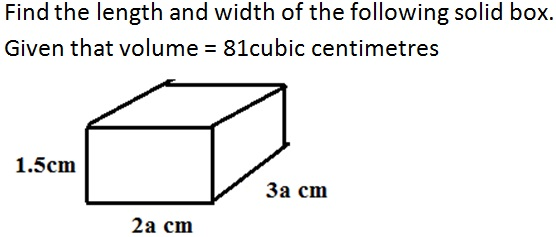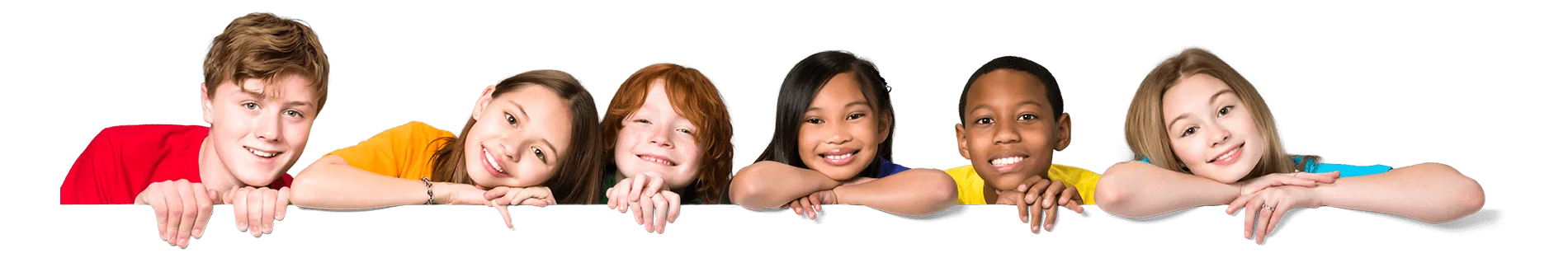Latest Results:

# 11plus 2d And 3d Shapes sample papers

Solve this Question

####1. 45° 2. 60° 3. 100° 4. 135°

Solve this Question

#### If we join two squares side by side, then what is the new shape of the figure?

 1. Rectangle 2. Square 3. Rhombus 4. Parallelogram

Solve this Question

#### John makes a Sandwich with two square-shaped slices of bread. The Sandwich is cut from one corner to the opposite corner (diagonal). What are the shapes of the slices that have been cut?

 1. Circles 2. Rectangles 3. Squares 4. Triangles

Solve this Question

#### In a triangle, the sum of two interior angles is 150 deg. Find the third angle.

 1. 90 deg 2. 40 deg 3. 30 deg 4. 20 deg

####